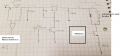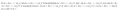# Energy and current in an inductor

#### nikina125

Joined Mar 24, 2019
2
Okay, stuff like that happens.

Now let's consider your lack of using units.

The final current in the inductor is not 5. It is 5 A. Because you didn't include the units, you messed up and said that the current (though you thought it was the initial current) was 5.0 mA. That's an error of three orders of magnitude!

Nearly all physical quantities have units and those units are an integral part of the quantity. It is meaningless to say that someone is 72 tall or that they weigh 100. Those give you zero information about the quantities described. You can't even make comparisons with them. Is someone that is 72 taller or shorter than someone that is 32? You have zero idea.

Beyond that, if you religiously track your units throughout your work, then you will find that you will catch most (not all) of your mistakes shortly after you make them instead of hours later (or not at all). In the academic realm this means you lose points unnecessarily. In the real world it means things get damaged and people get injured and killed unnecessarily.

Sadly, the people that write text books almost universally come from a purely academic background with little to no industry experience. As a result they have the academic mindset and are very sloppy with units (even when they've gone to lengths to say how important they are -- but they really only mean that it's important on the final answer). This attitude then infects the vast majority of students learning from those texts.

Consider the equation you started with (and that I'm guessing was given to you as is)

i = 5.0(1 - e^(-5000t))

This tells us nothing about the magnitude of the current -- is it 5 nA, 5 mA, 5 A, 5 kA? Any of those might be reasonable depending on the specifics.

This tells us nothing about the time scale of the decay -- is t in microseconds, milliseconds, seconds, hours? Again, any of those might be reasonable depending on the specifics.

So we are left with having to guess -- and engineering is NOT about guessing (at least not about this kind of stuff).

I'm guessing (see -- having to guess, which is major-time not good) that this is 5 A and that the time is in seconds.

Since the argument to any transcendental function has to be dimensionless, that means that the 5000 has units of inverse-seconds. Thus this equation should be

$$i(t) \; = \; 5.0 \, A \, \(1 \; - \; e^{-5000 \, s^{-1} \; t}$$
\)

In addition to being correct, by having the units we gain the option to write things in a manner that is easier for us to comprehend, such as:

$$i(t) \; = \; 5.0 \, A \, \(1 \; - \; e^{\frac{-t}{200 \, \mu s}}$$
\)

Now we can see immediately that we are dealing with a system that has a 200 μs time constant. If someone tells us that t = 1.2 ms we don't have to guess whether, perhaps, the t in the equation was supposed to be in milliseconds or not. Give me a time in picosecond or minutes and I can unambiguously tell you what the value of the exponent is in that equation.

I can also do the math without having to go to base units, which tend to be either so big or so small that humans are more likely to make mistakes. For instance, if you want the voltage across the inductor, then

$$v(t) \; = \; L \frac{di(t)}{dt} v(t) \; = \; 2.0 \, mH \frac{d}{dt}\(5.0 \, A \, \(1 \; - \; e^{\frac{-t}{200 \, \mu s}}$$ \)
v(t) \; = \; $$5.0 \cdot 2.0$$ \, mH \cdot A \frac{d}{dt}$$1 \; - \; e^{\frac{-t}{200 \, \mu s}}$$
v(t) \; = \; 10.0 \, mH \cdot A $$- \(\frac{-1}{200 \, \mu s}$$ \) e^{\frac{-t}{200 \, \mu s}}
v(t) \; = \; \frac{10.0}{200} \, \frac{mH \cdot A}{\mu s} \, e^{\frac{-t}{200 \, \mu s}}
v(t) \; = \; \frac{10.0}{200} \, \frac{1000 \, m}{1} \, \frac{mH \cdot A}{\mu s} \, e^{\frac{-t}{200 \, \mu s}}
v(t) \; = \; 50.0 \, \frac{m \cdot mH \cdot A}{\mu s} \, e^{\frac{-t}{200 \, \mu s}}
v(t) \; = \; 50.0 \, \frac{H \cdot A}{s} \, e^{\frac{-t}{200 \, \mu s}}
\)

Note that m·m cancels μ since (1 milli)(1 milli) = (1 micro).

From the constitutive equation for inductance itself we see that

$$1 \, H \; = \; 1 \, \frac{V \cdot s}{A}$$

Hence

$$v(t) \; = \; 50.0 \, \frac{H \cdot A}{s} \, e^{\frac{-t}{200 \, \mu s}} v(t) \; = \; 50.0 \, \frac{H \cdot A}{s} \, \frac{V \cdot s}{H \cdot A} \, e^{\frac{-t}{200 \, \mu s}} v(t) \; = \; 50.0 \, V \, e^{\frac{-t}{200 \, \mu s}}$$

And if the units hadn't worked out to a voltage at the end, we would have known that we had made a mistake somewhere along the way and gone and tracked it down. This is MUCH better than just taking on a V onto the end of the final answer because that is what we want the units to be.

Note that I did the above in much more detail than is normally needed just to show how it all works out.

#### nikina125

Joined Mar 24, 2019
2
Hi WBahn,
I am trying to understand how to measure energy stored in a 2.44mH. I am trying to measure system current when the valve is actuated. The valve has an inductor inside. Specs of inductor: 2.44mH @ 20KHz with a Q = 1.2. When I measure the system current when valve is actuated the current measured with the current probe is lower by 180mA or so than what It should be and I am trying to understand why.
Is it possible that a 2.44mA inductor is storing 180mA and releasing it just to the valve during the activation cycle. Could it be this the reason why the sense resistor that measures the system current doesn't see the total current drawn when the valve is actuated?
Circuit shown below:please let me know what you think.

I was trying to understand the explanation you were given before about how to measure or quantify the energy stored in an inductor but the formulas are all messed up. Is there a way to fix all these equation to better understand your explanation?Thanks!

#### Wendy

Joined Mar 24, 2008
22,174
@nikina125 I almost deleted all your posts. Next time start a new thread.

#### WBahn

Joined Mar 31, 2012
26,301
It would be best to start your own thread and reference this one.

The LaTeX rendering engines for some of the browsers changed a while back and won't render code that they should and that they used to. Try a different browser. I'm not going to spend hours figuring out how to get every browser to work like it should and then edit thousands of posts to update them.